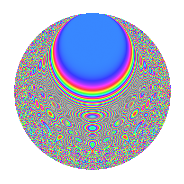# Properties

 Label 731.2.nLevel 731 Weight 2 Character orbit n Rep. character $$\chi_{731}(208,\cdot)$$ Character field $$\Q(\zeta_{12})$$ Dimension 256 Newforms 1 Sturm bound 132 Trace bound 0

# Related objects

## Defining parameters

 Level: $$N$$ = $$731 = 17 \cdot 43$$ Weight: $$k$$ = $$2$$ Character orbit: $$[\chi]$$ = 731.n (of order $$12$$ and degree $$4$$) Character conductor: $$\operatorname{cond}(\chi)$$ = $$731$$ Character field: $$\Q(\zeta_{12})$$ Newforms: $$1$$ Sturm bound: $$132$$ Trace bound: $$0$$

## Dimensions

The following table gives the dimensions of various subspaces of $$M_{2}(731, [\chi])$$.

Total New Old
Modular forms 272 272 0
Cusp forms 256 256 0
Eisenstein series 16 16 0

## Trace form

 $$256q - 6q^{3} - 264q^{4} + 2q^{5} - 2q^{6} + O(q^{10})$$ $$256q - 6q^{3} - 264q^{4} + 2q^{5} - 2q^{6} + 2q^{10} + 4q^{11} + 8q^{12} - 8q^{13} - 6q^{14} + 248q^{16} - 2q^{17} + 16q^{18} - 14q^{20} - 16q^{21} - 4q^{22} + 8q^{23} + 12q^{24} - 12q^{27} - 14q^{28} + 2q^{29} + 8q^{30} - 24q^{31} + 20q^{33} + 16q^{34} + 40q^{35} + 18q^{37} + 8q^{38} + 36q^{39} - 10q^{40} + 8q^{41} - 80q^{44} - 4q^{45} + 2q^{46} + 24q^{47} + 24q^{48} + 92q^{50} - 20q^{51} + 4q^{52} - 88q^{54} - 80q^{55} + 60q^{56} - 44q^{57} + 34q^{58} - 8q^{61} + 24q^{62} - 26q^{63} - 200q^{64} - 8q^{65} + 44q^{67} - 58q^{68} + 40q^{69} - 26q^{71} - 48q^{72} + 36q^{73} + 90q^{74} - 156q^{75} - 24q^{78} + 22q^{79} + 30q^{80} + 132q^{81} + 156q^{82} - 160q^{84} - 28q^{85} + 52q^{86} + 28q^{88} - 20q^{89} + 28q^{90} + 34q^{91} - 70q^{92} + 40q^{95} - 16q^{96} - 92q^{98} + 30q^{99} + O(q^{100})$$

## Decomposition of $$S_{2}^{\mathrm{new}}(731, [\chi])$$ into irreducible Hecke orbits

Label Dim. $$A$$ Field CM Traces $q$-expansion
$$a_2$$ $$a_3$$ $$a_5$$ $$a_7$$
731.2.n.a $$256$$ $$5.837$$ None $$0$$ $$-6$$ $$2$$ $$0$$